Function Repository Resource:

# FindCanonicalHypergraphIsomorphism

Find a canonical isomorphism between hypergraphs

Contributed by: Nikolay Murzin
 ResourceFunction["FindCanonicalHypergraphIsomorphism"][hg1, hg2] find a canonical isomorphism between hypergraphs hg1 and hg2 if it exists. ResourceFunction["FindCanonicalHypergraphIsomorphism"][hg] find an isomorphism from hypergraph hg to its canonical representation.

## Details and Options

Canonicalization follows the definition and implementation of the resource function CanonicalHypergraph.
ResourceFunction["FindCanonicalHypergraphIsomorphism"] returns an association with vertex replacements, similar to FindGraphIsomorphism.
With "IncludePermutation"True, a permutation of hyperedges is also included in output as {permutation, isomorphism}.

## Examples

### Basic Examples (3)

Find a canonical isomorphism between two hypergraphs:

 In:=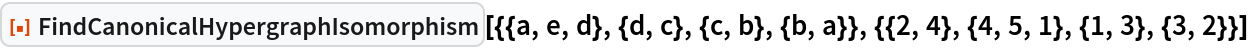Out=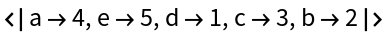Find an isomorphism to hypergraph's canonical representation:

 In:=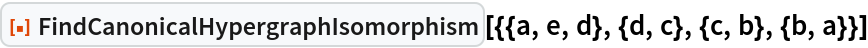Out=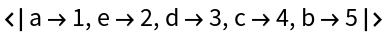Transform from one hypergraph to another if isomorphism exists:

 In:=In:=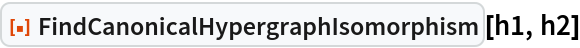Out=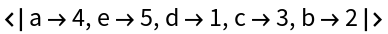In:=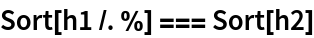Out=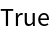### Scope (1)

If no isomorphism exists, \$Failed is returned:

 In:=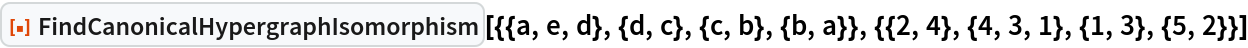Out=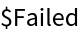### Options (1)

#### IncludePermutation (1)

Include a permutation of hyperedges in the output:

 In:=In:=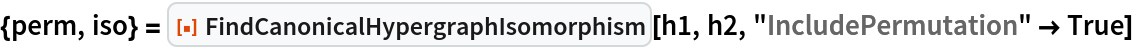Out=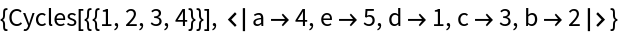In:=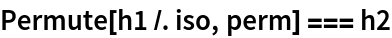Out=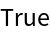### Properties and Relations (1)

The result of the resource function CanonicalHypergraph can be reproduced by combining an isomorphism with a permutation:

 In:=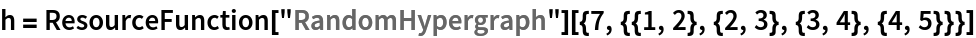Out=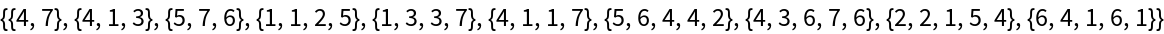In:=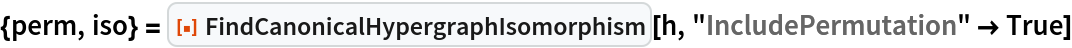Out=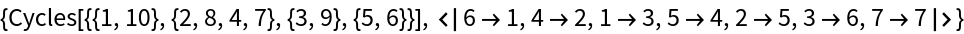In:=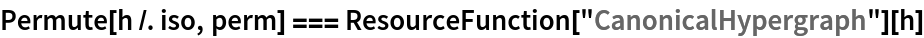Out=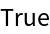## Version History

• 1.0.0 – 09 May 2022